Chemistry

# Chapter 19

ChemistryChapter 19

1.

(a) Sc: [Ar]4s23d1; (b) Ti: [Ar]4s23d2; (c) Cr: [Ar]4s13d5; (d) Fe: [Ar]4s23d6; (e) Ru: [Kr]5s24d6

3.

(a) La: [Xe]6s25d1, La3+: [Xe]; (b) Sm: [Xe]6s24f6, Sm3+: [Xe]4f5; (c) Lu: [Xe]6s24f145d1, Lu3+: [Xe]4f14

5.

Al is used because it is the strongest reducing agent and the only option listed that can provide sufficient driving force to convert La(III) into La.

7.

Mo

9.

The CaSiO3 slag is less dense than the molten iron, so it can easily be separated. Also, the floating slag layer creates a barrier that prevents the molten iron from exposure to O2, which would oxidize the Fe back to Fe2O3.

11.

2.57%

13.

0.167 V

15.

E° = −0.6 V, E° is negative so this reduction is not spontaneous. E° = +1.1 V

17.

(a) $Fe(s)+2H3O+(aq)+SO42−(aq)⟶Fe2+(aq)+SO42−(aq)+H2(g)+2H2O(l);Fe(s)+2H3O+(aq)+SO42−(aq)⟶Fe2+(aq)+SO42−(aq)+H2(g)+2H2O(l);$ (b) $FeCl3(aq)+3Na+(aq)+3OH−(aq)⟶Fe(OH)3(s)+3Na+(aq)+3Cl+(aq);FeCl3(aq)+3Na+(aq)+3OH−(aq)⟶Fe(OH)3(s)+3Na+(aq)+3Cl+(aq);$ (c) $Mn(OH)2(s)+2H3O+(aq)+2Br−(aq)⟶Mn2+(aq)+2Br−(aq)+4H2O(l);Mn(OH)2(s)+2H3O+(aq)+2Br−(aq)⟶Mn2+(aq)+2Br−(aq)+4H2O(l);$ (d) $4Cr(s)+3O2(g)⟶2Cr2O3(s);4Cr(s)+3O2(g)⟶2Cr2O3(s);$ (e) $Mn2O3(s)+6H3O+(aq)+6Cl−(aq)⟶2MnCl3(s)+9H2O(l);Mn2O3(s)+6H3O+(aq)+6Cl−(aq)⟶2MnCl3(s)+9H2O(l);$ (f) $Ti(s)+xsF2(g)⟶TiF4(g)Ti(s)+xsF2(g)⟶TiF4(g)$

19.

(a)
$Cr2(SO4)3(aq)+2Zn(s)+2H3O+(aq)⟶2Zn2+(aq)+H2(g)+2H2O(l)+2Cr2+(aq)+3SO42−(aq);Cr2(SO4)3(aq)+2Zn(s)+2H3O+(aq)⟶2Zn2+(aq)+H2(g)+2H2O(l)+2Cr2+(aq)+3SO42−(aq);$ (b) $4TiCl3(s)+CrO42−(aq)+8H+(aq)⟶4Ti4+(aq)+Cr(s)+4H2O(l)+12Cl−(aq);4TiCl3(s)+CrO42−(aq)+8H+(aq)⟶4Ti4+(aq)+Cr(s)+4H2O(l)+12Cl−(aq);$ (c) In acid solution between pH 2 and pH 6, $CrO42−CrO42−$ forms $HCrO4−,HCrO4−,$ which is in equilibrium with dichromate ion. The reaction is $2HCrO4−(aq)⟶Cr2O72−(aq)+H2O(l).2HCrO4−(aq)⟶Cr2O72−(aq)+H2O(l).$ At other acidic pHs, the reaction is $3Cr2+(aq)+CrO42−(aq)+8H3O+(aq)⟶4Cr3+(aq)+12H2O(l);3Cr2+(aq)+CrO42−(aq)+8H3O+(aq)⟶4Cr3+(aq)+12H2O(l);$ (d) $8CrO3(s)+9Mn(s)⟶Δ4Cr2O3(s)+3Mn3O4(s);8CrO3(s)+9Mn(s)⟶Δ4Cr2O3(s)+3Mn3O4(s);$ (e) $CrO(s)+2H3O+(aq)+2NO3−(aq)⟶Cr2+(aq)+2NO3−(aq)+3H2O(l);CrO(s)+2H3O+(aq)+2NO3−(aq)⟶Cr2+(aq)+2NO3−(aq)+3H2O(l);$ (f) $CrCl3(s)+3NaOH(aq)⟶Cr(OH)3(s)+3Na+(aq)+3Cl−(aq)CrCl3(s)+3NaOH(aq)⟶Cr(OH)3(s)+3Na+(aq)+3Cl−(aq)$

21.

(a) $3Fe(s)+4H2O(g)⟶Fe3O4(s)+4H2(g);3Fe(s)+4H2O(g)⟶Fe3O4(s)+4H2(g);$ (b) $3NaOH(aq)+Fe(NO3)3(aq)→H2OFe(OH)3(s)+3Na+(aq)+3NO3−(aq);3NaOH(aq)+Fe(NO3)3(aq)→H2OFe(OH)3(s)+3Na+(aq)+3NO3−(aq);$ (c) $MnO4−+5Fe2++8H+⟶Mn2++5Fe3+4H2O;MnO4−+5Fe2++8H+⟶Mn2++5Fe3+4H2O;$ (d) $Fe(s)+2H3O+(aq)+SO42−(aq)⟶Fe2+(aq)+SO42−(aq)+H2(g)+2H2O(l);Fe(s)+2H3O+(aq)+SO42−(aq)⟶Fe2+(aq)+SO42−(aq)+H2(g)+2H2O(l);$ (e) $4Fe2+(aq)+O2(g)+4HNO3(aq)⟶4Fe3+(aq)+2H2O(l)+4NO3−(aq);4Fe2+(aq)+O2(g)+4HNO3(aq)⟶4Fe3+(aq)+2H2O(l)+4NO3−(aq);$ (f) $FeCO3(s)+2HClO4(aq)⟶Fe(ClO4)2(aq)+H2O(l)+CO2(g);FeCO3(s)+2HClO4(aq)⟶Fe(ClO4)2(aq)+H2O(l)+CO2(g);$ (g) $3Fe(s)+2O2(g)⟶ΔFe3O4(s)3Fe(s)+2O2(g)⟶ΔFe3O4(s)$

23.

$Ag+(aq)+CN−(aq)⟶AgCN(s)Ag+(aq)+CN−(aq)⟶AgCN(s)$
$Ag+(aq)+2CN−(aq)⟶[Ag(CN)2]−(aq)AgCN(s)+CN−(aq)⟶[Ag(CN)2]−(aq)Ag+(aq)+2CN−(aq)⟶[Ag(CN)2]−(aq)AgCN(s)+CN−(aq)⟶[Ag(CN)2]−(aq)$

25.

(a) Sc3+; (b) Ti4+; (c) V5+; (d) Cr6+; (e) Mn4+; (f) Fe2+ and Fe3+; (g) Co2+ and Co3+; (h) Ni2+; (i) Cu+

27.

(a) 4, [Zn(OH)4]2−; (b) 6, [Pd(CN)6]2−; (c) 2, [AuCl2]; (d) 4, [Pt(NH3)2Cl2]; (e) 6, K[Cr(NH3)2Cl4]; (f) 6, [Co(NH3)6][Cr(CN)6]; (g) 6, [Co(en)2Br2]NO3

29.

(a) [Pt(H2O)2Br2]:(b) [Pt(NH3)(py)(Cl)(Br)]: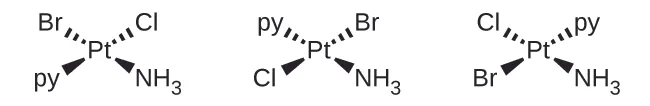(c) [Zn(NH3)3Cl]+ :(d) [Pt(NH3)3Cl]+ :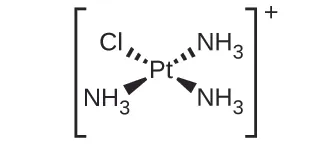(e) [Ni(H2O)4Cl2]: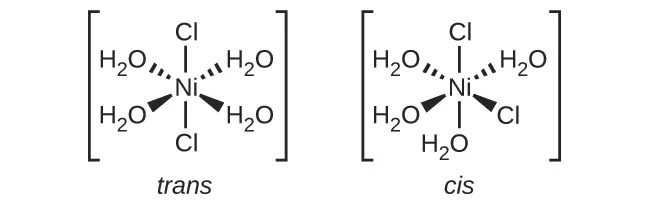(f) [Co(C2O4)2Cl2]3−: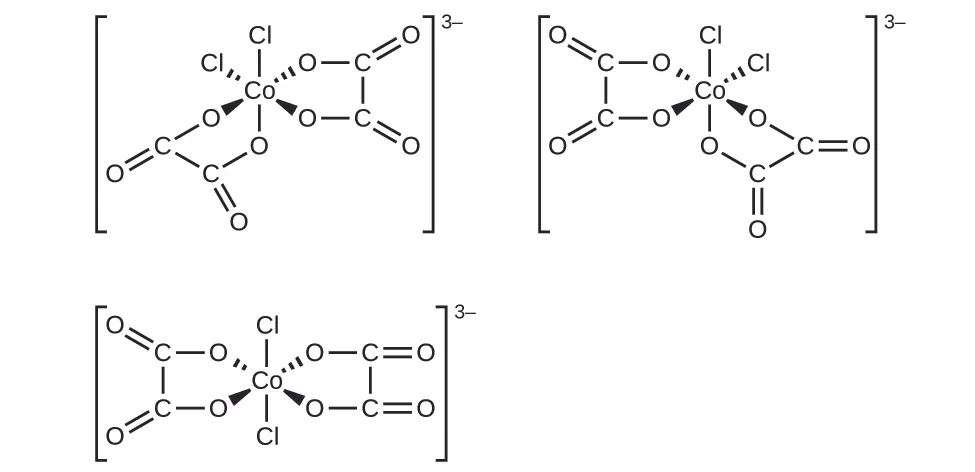31.

(a) tricarbonatocobaltate(III) ion; (b) tetraaminecopper(II) ion; (c) tetraaminedibromocobalt(III) sulfate; (d) tetraamineplatinum(II) tetrachloroplatinate(II); (e) tris-(ethylenediamine)chromium(III) nitrate; (f) diaminedibromopalladium(II); (g) potassium pentachlorocuprate(II); (h) diaminedichlorozinc(II)

33.

(a) none; (b) none; (c) The two Cl ligands can be cis or trans. When they are cis, there will also be an optical isomer.

35.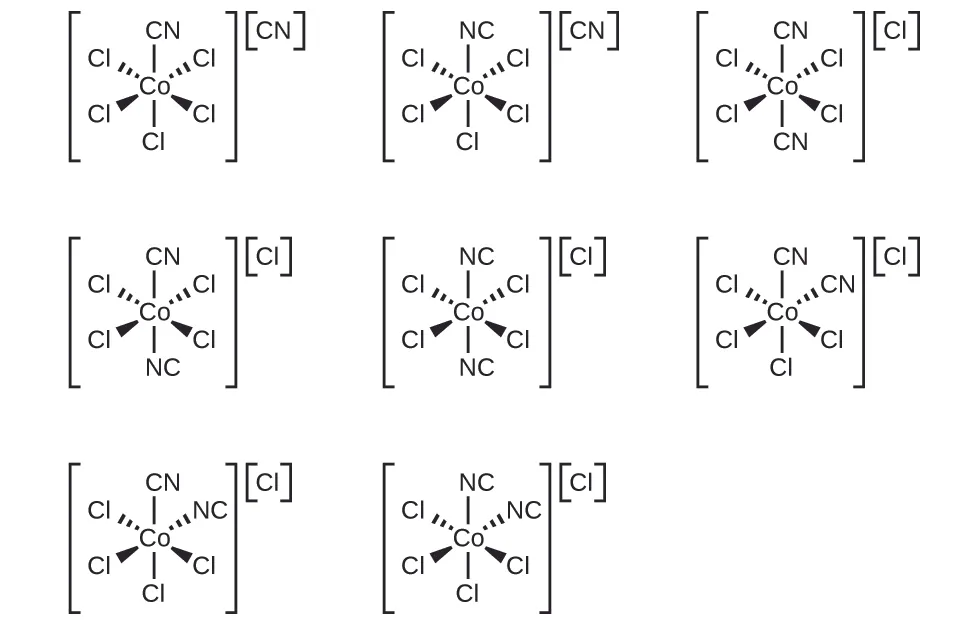37.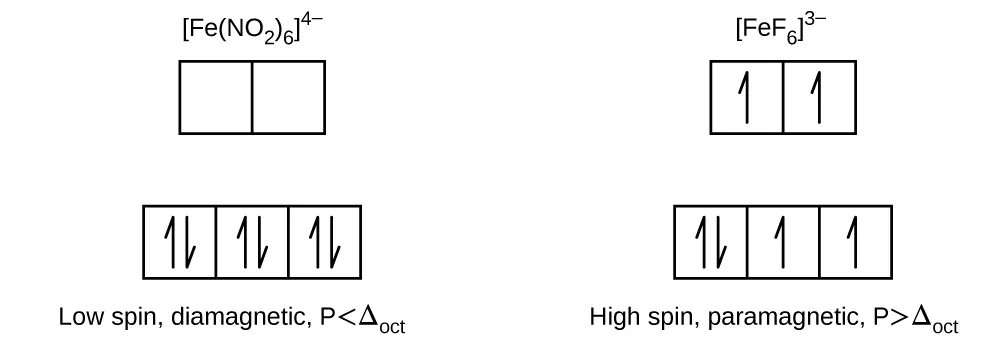39.

[Co(H2O)6]Cl2 with three unpaired electrons.

41.

(a) 4; (b) 2; (c) 1; (d) 5; (e) 0

43.

(a) [Fe(CN)6]4−; (b) [Co(NH3)6]3+; (c) [Mn(CN)6]4−

45.

The complex does not have any unpaired electrons. The complex does not have any geometric isomers, but the mirror image is nonsuperimposable, so it has an optical isomer.

47.

No. Au+ has a complete 5d sublevel.

Order a print copy

As an Amazon Associate we earn from qualifying purchases.